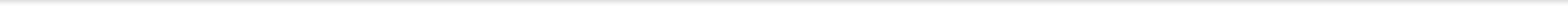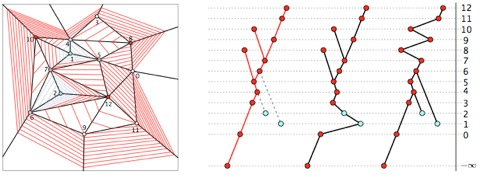# I/O-Efficient Contour Queries on TerrainsPankaj K. Agarwal, Thomas Mølhave, and Bardia Sadri

Given a terrain in the form of a triangulation of the plane with a height function associated to vertices (and linearly interpolated within the edges and triangles), we investigate the problem of I/O-efficiently answering contour queries: Given a regular height value l and a triangle t of the terrain which intersects the level-set at height l as input, the output of the query is the list of the edges of the connected component of the level-set at height l that intersect t, reported in clockwise or counter-clockwise order. Notice that contour queries are different from level-set queries in that only one contour out of all those in the corresponding level set is required to be reported. We present an I/O-efficient data structure of linear size that can be used to answer a contour query in O(log
B N + T/B) I/Os, where T is the number of triangles in the contour. The data structure can be constructed using O(sort(N)) I/Os.

ACM-SIAM Symposium on Discrete Mathematics (
SODA), 2011 [PDF]